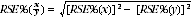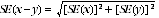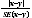4130.0.55.001 - Housing Occupancy and Costs, Australia, 2005-06
Latest ISSUE Released at 11:30 AM (CANBERRA TIME) 31/10/2007
 Page tools: .mffeedback,#pjs { display :none; } Enable Javascript to Print PagesPrint AllEmail NotificationRSSSearch this Product Explanatory Notes Glossary Abbreviations ABS Housing Statistics (Appendix 1) Sampling Variability (Appendix 2) Imputed rent for owner-occupied dwellings (Appendix 3) APPENDIX 2 SAMPLING VARIABILITY INTRODUCTION The estimates in this publication are based on information obtained from the occupants of a sample of dwellings. Therefore, the estimates are subject to sampling variability and may differ from the figures that would have been produced if information had been collected for all dwellings. One measure of the likely difference is given by the standard error (SE), which indicates the extent to which an estimate might have varied because only a sample of dwellings was included. There are about two chances in three that the sample estimate will differ by less than one SE from the figure that would have been obtained if all dwellings had been included, and about 19 chances in 20 that the difference will be less than two SEs. Another measure of the likely difference is the relative standard error (RSE), which is obtained by expressing the SE as a percentage of the estimate. For estimates of population sizes, the size of the SE generally increases with the level of the estimate, so that the larger the estimate the larger the SE. However, the larger the sampling estimate the smaller the SE in percentage terms (RSE). Thus, larger sample estimates will be relatively more reliable than smaller estimates. In the tables in this publication, only estimates with RSEs of 25% or less are considered reliable for most purposes. Estimates with RSEs greater than 25% but less than or equal to 50% are annotated by an asterisk to indicate they are subject to high SEs and should be used with caution. Estimates with RSEs of greater than 50%, annotated by a double asterisk, are considered too unreliable for general use and should only be used to aggregate with other estimates to provide derived estimates with RSEs of 25% or less. Space does not allow for the separate indication of the SE of all the estimates in this publication. RSEs for all tables are provided on the ABS web site (see Statistics: Access to all ABS products and statistics, Statistics by Catalogue Number, 41, 4130.0.55.001 - Housing Occupancy and Costs, Australia, 2005-06). The RSEs have been derived using the group jackknife method. COMPARATIVE ESTIMATES Proportions and percentages Proportions and percentages, which are formed from the ratio of two estimates, are also subject to sampling errors. The size of the error depends on the accuracy of both the numerator and the denominator. For proportions where the denominator is an estimate of the number of households in a grouping and the numerator is the number of households in a sub-group of the denominator group, the formula for the RSE is given by:Differences between estimates The difference between survey estimates is also subject to sampling variability. An approximate SE of the difference between two estimates (x-y) may be calculated by the formula:This approximation can generally be used whenever the estimates come from different samples, such as two estimates from different years or two estimates for two non-intersecting subpopulations in the one year. If the estimates come from two populations, one of which is a subpopulation of the other, the standard error is likely to be lower than that derived from this approximation, but there is no straightforward way of estimating how much lower. SIGNIFICANCE TESTING Statistical significance testing can be undertaken to determine whether it is likely that there is a difference between two estimates from different samples. The standard error for the difference between two estimates can be calculated using the formula in the paragraph above. This standard error is used to calculate the following test statistic:If the value of this test statistic is greater than 1.96 then there are 19 chances in 20 that there is a real difference in the two populations with respect to that characteristic. Otherwise, it cannot be stated with confidence that there is a real difference between the populations. Document Selection These documents will be presented in a new window.